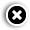# How EPF interest is calculated: All you need to know

## While the EPF contribution is deposited every month, the interest is calculated at the end of the year.The interest is calculated by multiplying the monthly balance with interest rate.

The Employees' Provident Fund Organisation (EPFO) on Thursday, March 4, kept the interest rate unchanged from last year at 8.5 per cent for fiscal 2020-21.

While an employee contributes 12 per cent of basic salary and dearness allowance to employees provident fund (EPF), employer contributes 8.33 per cent towards Employees' Pension Scheme (EPS) and 3.67 per cent to employees' EPF. Here is how the interest rate for EPF is calculated:

While the EPF contribution is deposited every month, the interest is calculated at the end of the year. EPFO calculates monthly closing balance every month and then the interest is calculated. The interest is calculated by multiplying the monthly balance with interest rate. Since the interest rate is 8.50 per cent annually, it will be divided by 12 to calculate the monthly interest (8.5/12 gives 0.7083).

So, if the running balance at the end of a month is Rs 5,000, interest will be 5,000*0.007083. The interest is calculated in the same way at the end of each month.

On Thursday, EPFO's central board of trustees, who met in Srinagar, ratified the 8.5 per cent interest rate after assessing earnings and financial positions. The meeting was chaired by Labour and Employment Minister Santosh Kumar Gangwar.

Although the 8.5 per cent interest disbursement is the same as last fiscal, it will cheer 60 million (6 crore) subscribers, and anything less than the said rate could have been the lowest in a decade. The EPF interest rate stood at 8.65 per cent in FY19.

• Print
• COMMENT
##### JK Tyre ties up with CarDekho, AutoBrix for doorstep services